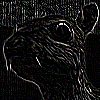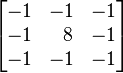# Workshop 2

• convert an index into column and row indices
• use the column and row values to do more than just change colours
• Learn about the kernel and convolusion

## The Workshop

• In the last workshop, we focused on each pixel, individually
• In this workshop, we will not only focus on a particular pixel, we will also be focusing on its neighbouring pixels
• We will be using something called a "kernel" to help us achieve some cool effects

## Converting an index into column and row indices

``````
var col = index % width;
var row = Math.floor(index / width);
``````

Sometimes, `col` (or column) and `row` are called `x` and `y`, respectively.

## Converting column and row indices into an array index

``````
var index = col + (row * width);
``````

## Blurring

Before we go ahead with the kernel, let's take some baby steps by first talking about blurring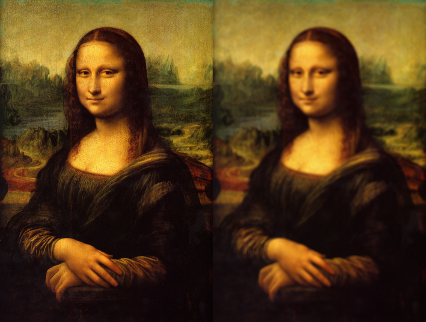## What blurring is

A best way to illustrate blurring is through shadowsThe penumbra is a simultaneous mixture of absence of light, and the presence thereof

## What blurring is (cont'd)

• similar idea for images
• blurring is an intended colour mixed with neighbouring light sources
• Remember: another way think of images---that our eyes see---as being a quasi-infinite array of light sources. If they mix, the images appear blurred

## Applying to digital images

• To blur an image, we iterate over every pixel, and we hollistically derive a value based on each pixel's neighbour
• a simple way to derive such value is to simply calculate the average
• Below is a simple blur applied to the Mona Lisa's Eye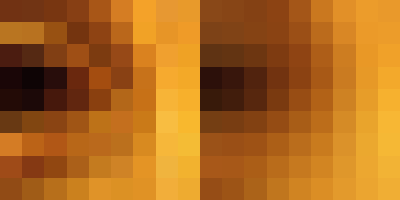## The Algorithm

This is for a simple box blur

``````
var kernelDimension = 3;
var kernelSize      = kernelDimension * kernelDimension;

for (var i = 0; i < pixels.length; i++) {
var col = index % width;
var row = Math.floor(index / height);

var accum = 0;

for (var x = 0; x < kernelDimension; x++) {
for (var y = 0; y < kernelDimension; y++) {
var lookupX = col + x - 1; if (lookupX < 0) { lookupX = 0; }
var lookupY = row + y - 1; if (lookupY < 0) { lookupY = 0; }

var index = lookupX + (lookupY * width);

accum += pixels[index];
}
}

pixels[i] = accum / kernelSize;
}
``````

This is just a demonstration. Actual code is different

## Another way to compute the pixel: multiply based on a kernel table

• the kernel is a square matrix of numbers. It has an odd number of rows and columns
• the pixel values are multiplied by the values in the kernel, and then added together, and the pixel is then set to their sum
• Below is an example kernel used for box blur

``````
[ 0.111, 0.111, 0.111,
0.111, 0.111, 0.111,
0.111, 0.111, 0.111 ]
``````

Question: why have all the values been set to 0.111?

## The Algorithm for our kernel

``````
var kernel =
[ 0.111, 0.111, 0.111,
0.111, 0.111, 0.111,
0.111, 0.111, 0.111 ]

var kernelDimension = 3;
var kernelSize      = kernelDimension * kernelDimension;

for (var i = 0; i < pixels.length; i++) {
var col = i % width;
var row = Math.floor(i / width);

var accum = 0;

for (var j = 0; j < kernelSize; j++) {
var x = j % kernelDimension;
var y = Math.floor(j / kermelDimension);

var lookupX = col + x - 1; if (lookupX < 0) { lookupX = 0; }
var lookupY = row + y - 1; if (lookupY < 0) { lookupY = 0; }

var index = lookupX + (lookupY * width);

accum += pixels[index] * kernel[j];
}

pixels[i] = accum;
}
``````

This is just a demonstration. Actual code is different

## Effects that can be achieved using the Kernel

Sharpen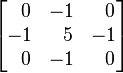## Effects that can be achieved using the Kernel

Box Blur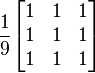## Effects that can be achieved using the Kernel

Gaussian Blur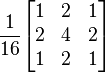## Effects that can be achieved using the Kernel

Edge Detection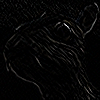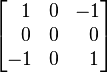## Effects that can be achieved using the Kernel

Edge Detection, another way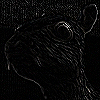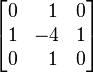## Effects that can be achieved using the Kernel

Edge Detection, yet another way